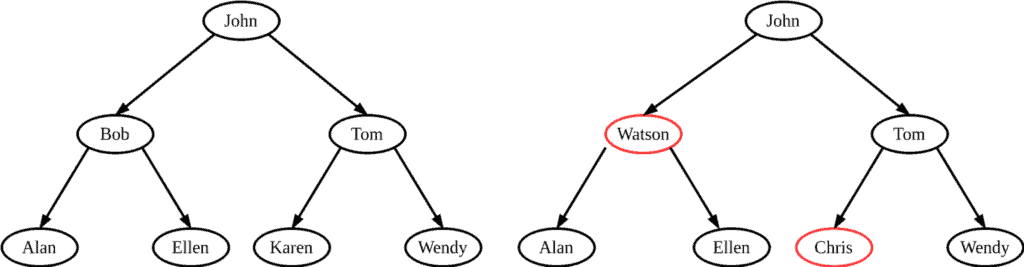## 1. Overview

In this tutorial, we’ll talk about the binary search tree (BST) data structure focusing more on the case where the keys in the nodes are represented by strings.

## 2. Introduction

A BST is a binary tree that satisfies the following properties for every node:

• The left subtree of the node contains only nodes with keys lesser than the node’s key
• The right subtree of the node contains only nodes with keys greater than the node’s key
• The left and right subtree each must also be a binary search tree.

In this article, we focus more on the case where the keys of the nodes are represented by strings and not numbers. In this case, we should first define the ordering of the strings.

Lexicographic ordering is defined as the order that each string that appears in a dictionary. To determine which string is lexicographically larger we compare the corresponding characters of the two strings from left to right. The first character where the two strings differ determines which string comes first. Characters are compared using the Unicode character set and all uppercase letters come before lowercase letters.

For example:

• apple < orange because ‘a’ comes before ‘o’
• Orange > orange because ‘O’ comes after ‘o’
• apple = apple because all letters are the same

Let’s take a look at two binary trees with strings:The left tree is a BST since it satisfies the above criterion while the right tree is not a BST because in the red nodes the criterion fails. ‘Watson’ is lexicographically larger than ‘John’ and ‘Chris’ is lexicographically smaller than ‘John’.

## 3. Basic Operations

As the name suggests, the most frequent operation in a BST with strings is searching for a specific string. Starting from the root we follow a downward path until we find the requested string.

For each node we encounter, we lexicographically compare the requested stringwith the node’s string. If, we continue the search in the left subtree since the BST property implies thatcould not be stored in the right subtree. Symmetrically, ifwe search the right subtree. The whole procedure is summarized in the above pseudocode:

``````

Other useful operations are to insert or delete a specific string from the BST. The data structure must be modified to reflect this change, but in such a way that the binary-search-tree property continues to hold. When inserting a new string, we basically search for the string in the tree using the BST-Search method. When we reach the NULL pointer (since the string is not present in the tree), we insert a node that contains the input string:

``

The process of deletion is slightly more intricate. There are three cases:

• If the node has no children, we simply remove it
• If the node has just one child, we replace the node with its child
• If the node has two children, we find its successor that lies in the right subtree and has no left child. Then, we replace the node with its successor

Let’s see the pseudocode:

``````

In order to move subtrees within the binary search tree, we define a subroutine TRANSPLANT, which replaces one subtree as a child of its parent with another subtree. When TRANSPLANT replaces the subtree rooted at nodewith the subtree rooted at node, node’s parent becomes node‘s parent, and’s parent ends up havingas its appropriate child.

The pseudocode for the TRANSPLANT function is as follows:## 4. Complexity Analysis

In a BST searching, insertion and deletion run in timetime whereis the height of the tree:## 5. Conclusion

In this article, we presented the basic operations of a BST that contains strings as keys.